# Matrices & Determinants Questions and Answers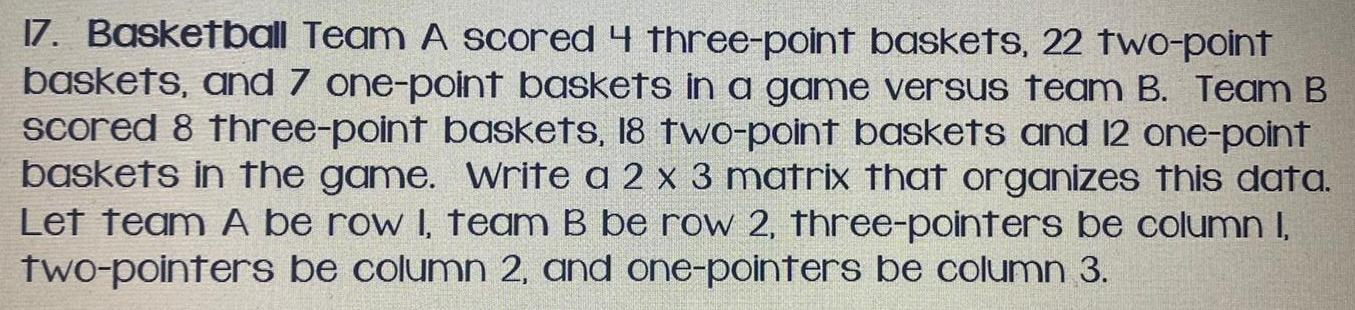Math
Matrices & Determinants
17. Basketball Team A scored 4 three-point baskets, 22 two-point baskets, and 7 one-point baskets in a game versus team B. Team B scored 8 three-point baskets, 18 two-point baskets and 12 one-point baskets in the game. Write a 2 x 3 matrix that organizes this data. Let team A be row I, team B be row 2, three-pointers be column I, two-pointers be column 2, and one-pointers be column 3.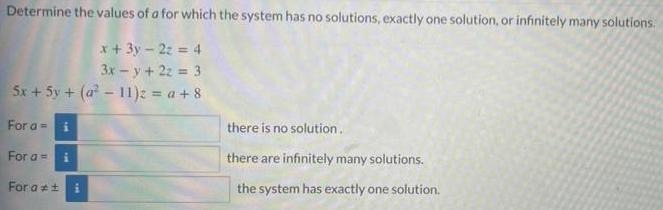Math
Matrices & Determinants
Determine the values of a for which the system has no solutions, exactly one solution, or infinitely many solutions. x + 3y - 2z = 4 3x - y + 2z = 3 5x + 5y + (a²-11)z = a +8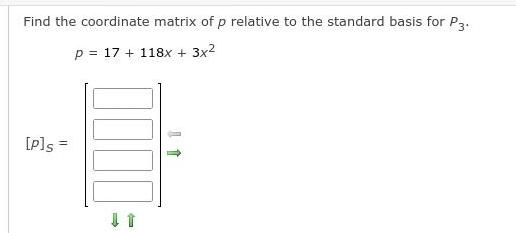Math
Matrices & Determinants
Find the coordinate matrix of p relative to the standard basis for P3. p = 17 + 118x + 3x²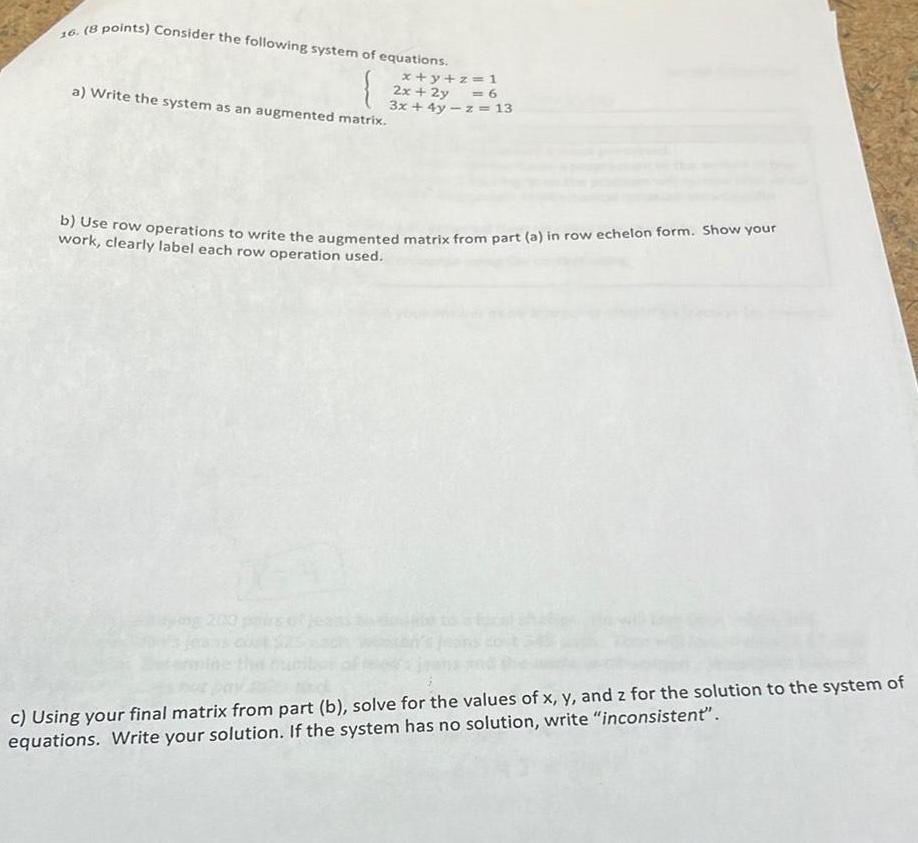Math
Matrices & Determinants
Consider the following system of equations. x+y+z=1 2x + 2y = 6 3x + 4y -z = 13 a) Write the system as an augmented matrix. buse row operations to write the augmented matrix from part (a) in row echelon form. Show your work, clearly label each row operation used. c) Using your final matrix from part (b), solve for the values of x, y, and z for the solution to the system of equations. Write your solution. If the system has no solution, write "inconsistent'".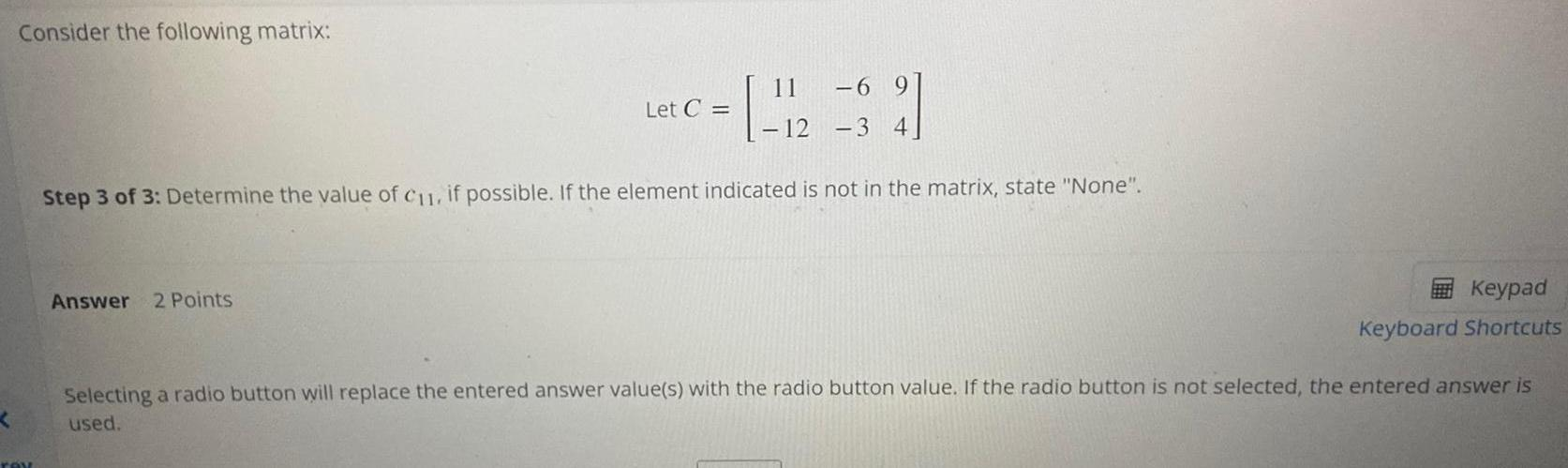Math
Matrices & Determinants
Consider the following matrix: Step 3 of 3: Determine the value of C11, if possible. If the element indicated is not in the matrix, state "None".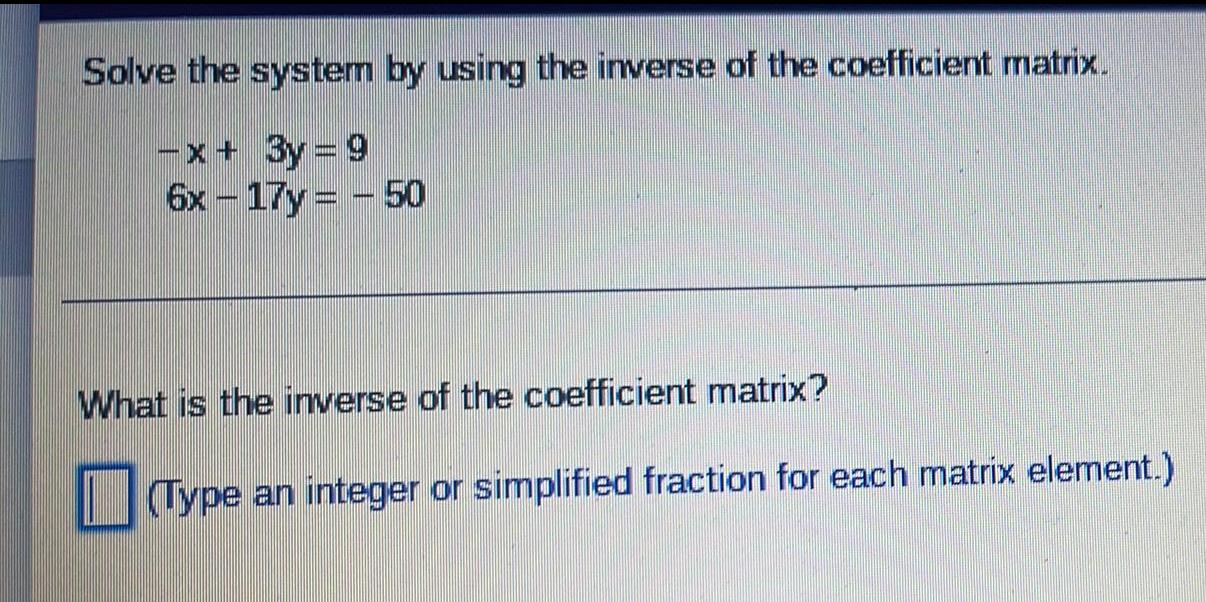Math
Matrices & Determinants
Solve the system by using the inverse of the coefficient matrix. -x+ 3y=9 6x-17y - 50 What is the inverse of the coefficient matrix? (Type an integer or simplified fraction for each matrix element.)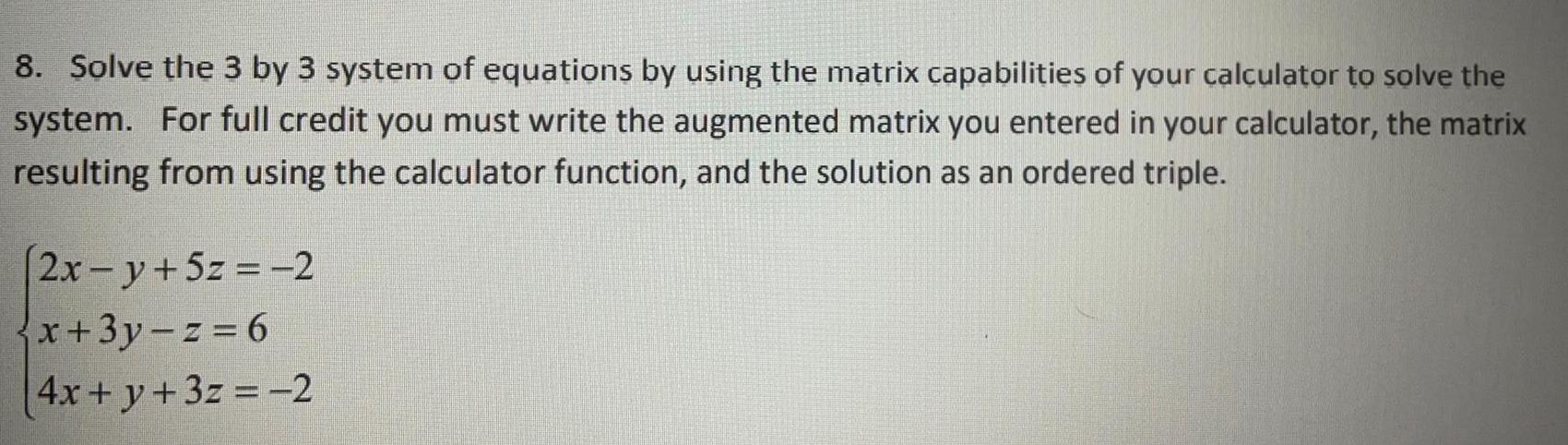Math
Matrices & Determinants
Solve the 3 by 3 system of equations by using the matrix capabilities of your calculator to solve the system. For full credit you must write the augmented matrix you entered in your calculator, the matrix resulting from using the calculator function, and the solution as an ordered triple. 2x -y + 5z = -2 x+3y-z=6 4x + y + 3z = -2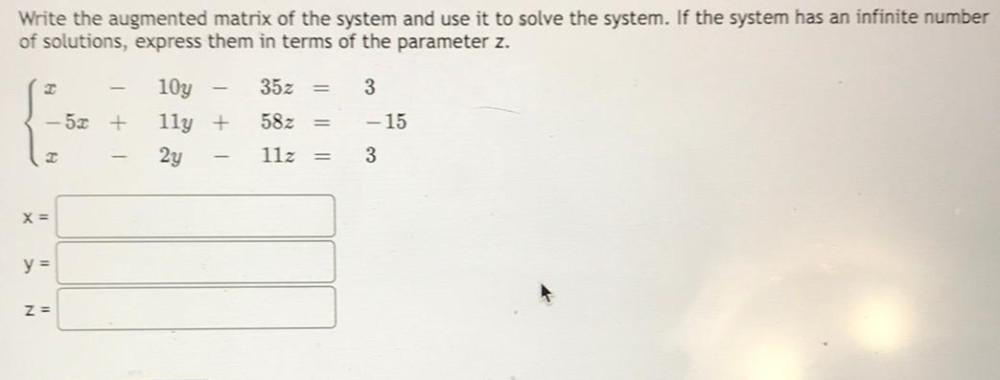Math
Matrices & Determinants
Write the augmented matrix of the system and use it to solve the system. If the system has an infinite number of solutions, express them in terms of the parameter z. x-10y-35z=3 -5x+11y+58z=-15 x-2y-11x=3 x=________________ y=________________ z=________________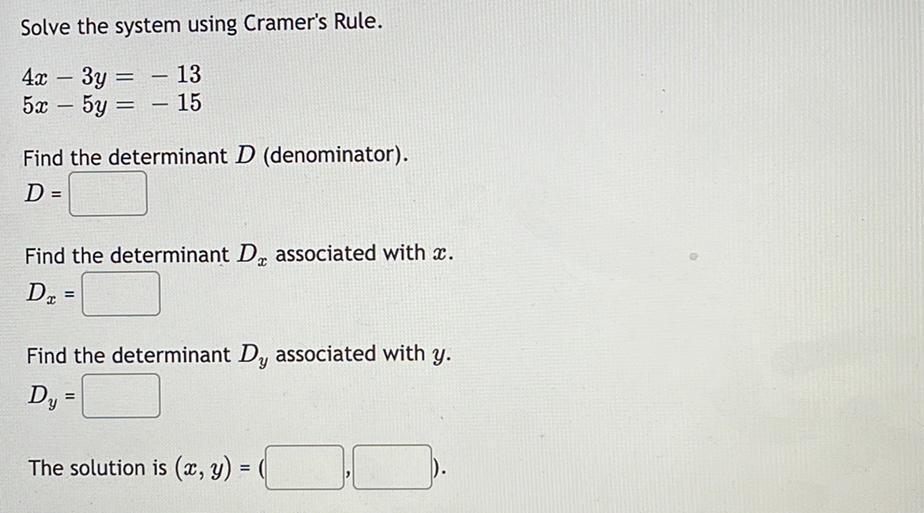Math
Matrices & Determinants
Solve the system using Cramer's Rule. 4x - 3y = -13 5x – 5y = -15 Find the determinant D (denominator). D = Find the determinant Dₓ associated with x. Dₓ = Find the determinant Dᵧ associated with y. Dᵧ= The solution is (x, y) =( , ).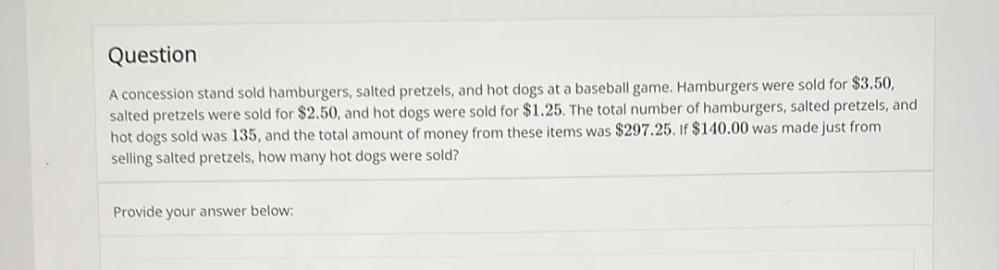Math
Matrices & Determinants
A concession stand sold hamburgers, salted pretzels, and hot dogs at a baseball game. Hamburgers were sold for \$3.50, salted pretzels were sold for \$2.50, and hot dogs were sold for \$1.25. The total number of hamburgers, salted pretzels, and hot dogs sold was 135, and the total amount of money from these items was \$297.25. If \$140.00 was made just from selling salted pretzels, how many hot dogs were sold?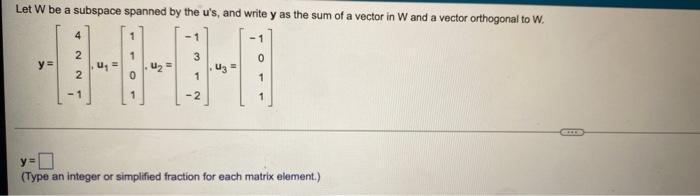Math
Matrices & Determinants
Let W be a subspace spanned by the u's, and write y as the sum of a vector in W and a vector orthogonal to W. y = 4 u₁ = 1 u₂ = -1 u₃ = -1 2 1 3 0 2 0 1 1 -1 1 -2 1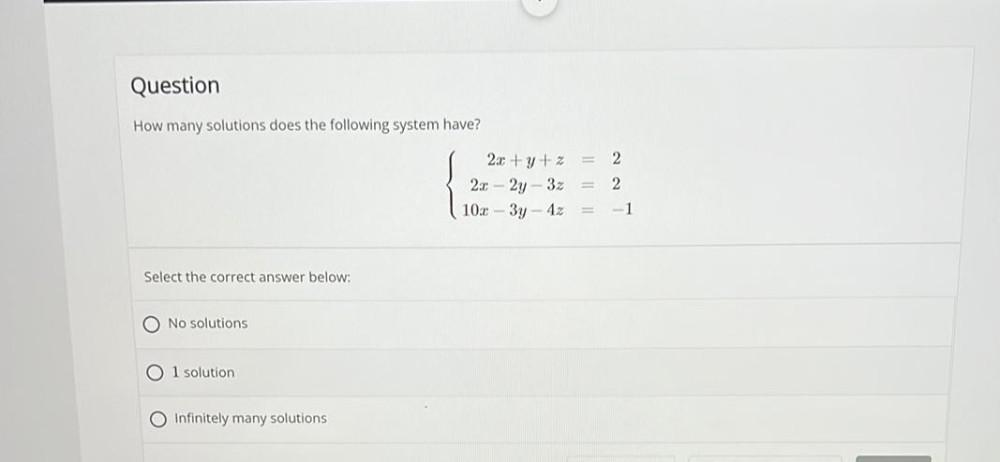Math
Matrices & Determinants
How many solutions does the following system have? 2x + y + z=2 2x - 2y - 3z=2 10x - 3y - 4z=-1 Select the correct answer below: a) No solutions b)l solution c)Infinitely many solutions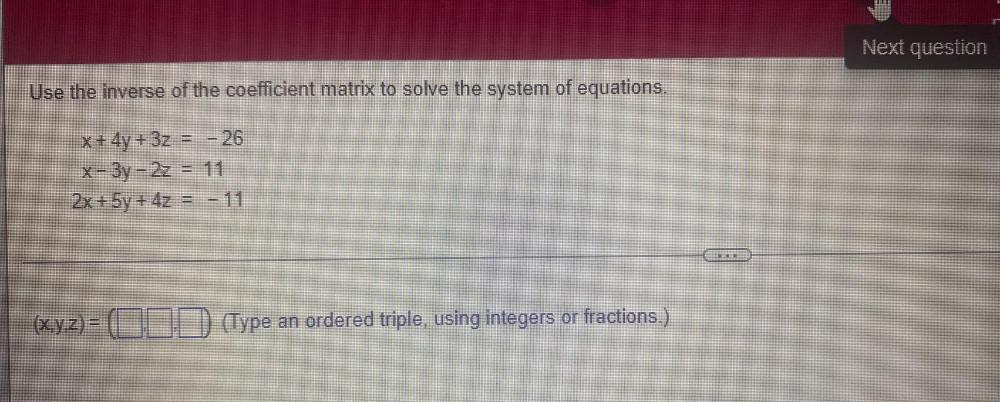Math
Matrices & Determinants
Use the inverse of the coefficient matrix to solve the system of equations. x + 4y + 3z = - 26 x - 3y - 2z = 11 2x + 5y + 4z = -11 (x,y,z)= (__,__,__)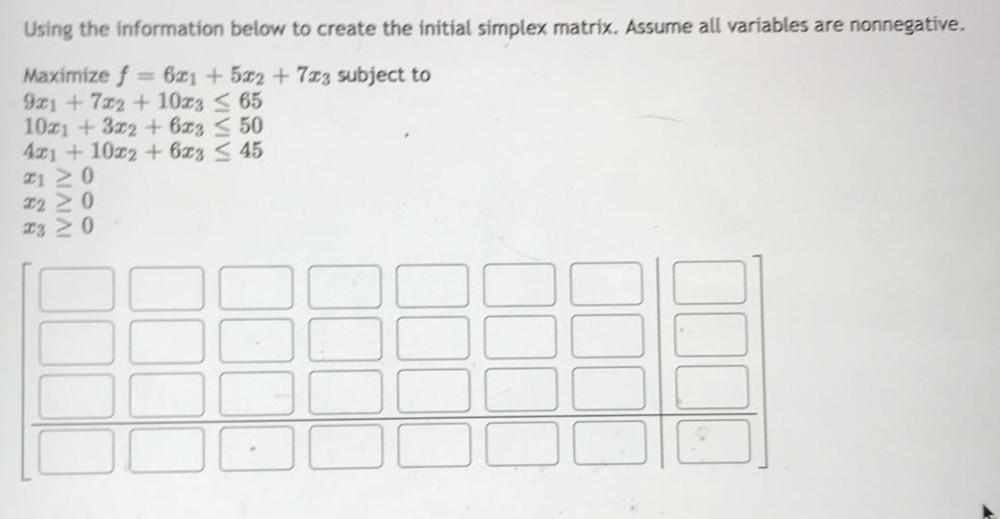Math
Matrices & Determinants
Using the information below to create the initial simplex matrix. Assume all variables are nonnegative. Maximize f = 6x₁ +5x₂ + 7x₃ subject to 9x₁ + 7x₂ + 10x₃ ≤ 65 10x₁ + 3x₂ + 6x₃ ≤ 50 4x₁ + 10x₂ + 6x₃ ≤ 45 x₁ ≥0 x₂ ≥ 0 x₃ ≥ 0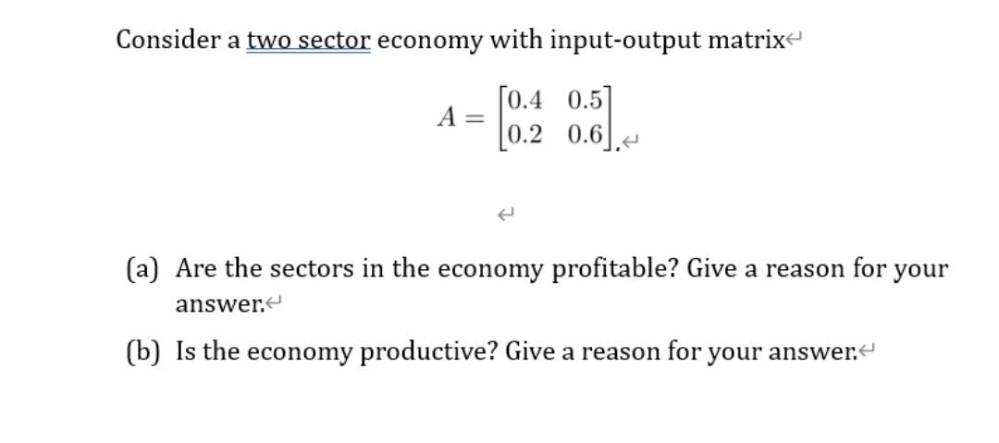Math
Matrices & Determinants
Consider a two sector economy with input-output matrix A= [0.4 0.5] [0.2 0.6] (a) Are the sectors in the economy profitable? Give a reason for your answer. (b) Is the economy productive? Give a reason for your answer.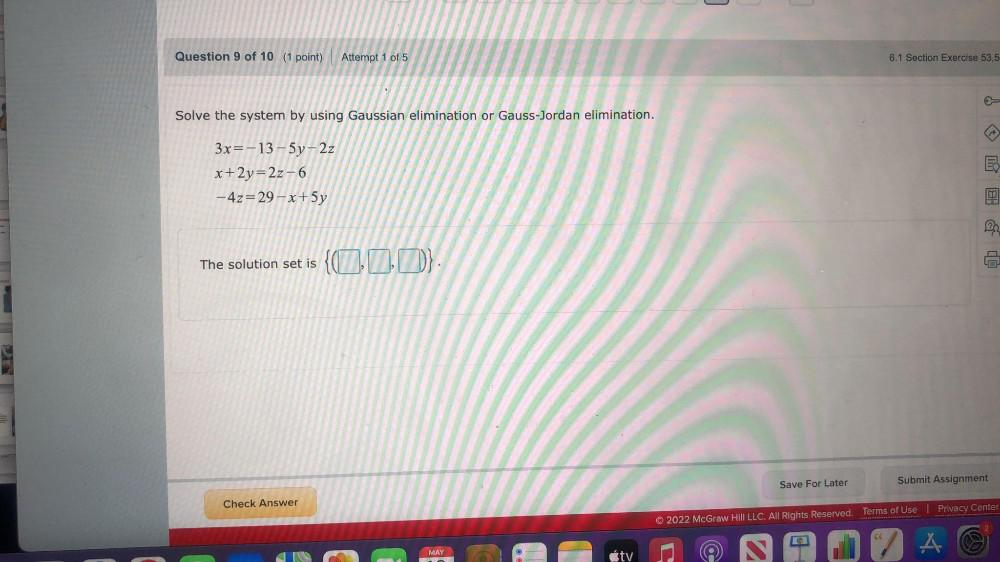Math
Matrices & Determinants
Solve the system by using Gaussian elimination or Gauss-Jordan elimination. 3x = - 13 - 5y - 2z x + 2y = 2z - 6 - 4z = 29 - x + 5y The solution set is {( )}